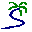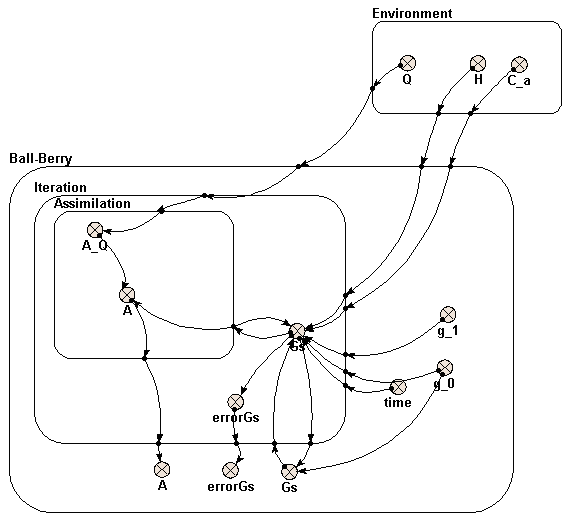# Model entry

Iterative solution to Ball-Berry stomatal conductance simultaneous equations (Simile V3+) - Model catalogue - Simulistics.com

 Search Simulistics Model catalogue Listed by keyword Listed by ID Listed by title Listed by date added

# Iterative solution to Ball-Berry stomatal conductance simultaneous equations (Simile V3+)

Model : ballberry1
Simile version : 3.1+
Keywords : Ecophysiology ; Stomatal conductance ; Evapotranspiration ; Technique ;

## Description

Here�s an example of a model with two simultaneous equations implemented in Simile. It is a version of the Ball-Berry stomatal conductance model where assimilation (photosynthesis) is a function of stomatal conductance and stomatal conductance is a function of assimilation.

Where there is a big change in stomatal conductance (Gs) more iterations are needed to reach a certain accuracy.

The iteration submodel is at time step #2. This and its contained submodels have a time step of 0.01 in the run control. The rest of the model has a time step of 1. The iteration thus runs 100 times for each time step of the container submodel.

## Files

### Model file

Click on the icon to download the model file. (You will need Simile to examine and run the model. A free evaluation version is available from the products page.)

Some browsers may attempt to display the model file, rather than open it in Simile; in this case, use the browser back button to return to this page, and use the context menu (invoked by right-clicking on the link) to save the target file to disk.ballberry1.sml

## Diagram## Equations

```Equations in Desktop

Equations in Assimilation

Variable   A : Assimation (umol CO2 m^-2 s^-1)
A = A_Q*last(Gs_0)
Where:
Gs_0=../Gs

Variable   A_Q : Assimilation light response curve
A_Q = graph(Q)
Where:
Q=../../../Environment/Q
Relationship of Assimilation with photon flux density (light) when stomatal conductance (Gs) is maximum

Equations in Environment

Variable   C_a : Carbon dioxide concentration (umol CO2 (mol air)^-1)
C_a = graph(time())
Typical diurnal curve in forest canopy

Variable   H : Relative humidity (proportion)
H = graph(time())
Typical diurnal graph (24 hour)

Variable   Q : Photon flux density (umol m^-2 s^-1)
Q = graph(time())
Graph for a sunny day (24 hours)

Equations in Iteration

Variable   Gs : Stomatal conductance (mol m^-2 s^-1)
Gs = if time()==time then Gs else g_0+g_1*A*H/C_a
Where:
A=Assimilation/A
time=../time
H=../../Environment/H
C_a=../../Environment/C_a
g_0=../g_0
g_1=../g_1
Gs=../Gs
Ball-Berry equation

Variable   errorGs
errorGs = Gs_0-last(Gs_0)
Where:
Gs_0=Gs

Equations in Ball-Berry

Variable   A
A = A
Where:
A=Iteration/Assimilation/A

Variable   Gs
Gs = if time()==0 then g_0 else last(Gs_0)
Where:
Gs_0=Iteration/Gs

Variable   errorGs
errorGs = last(errorGs_0)
Where:
errorGs_0=Iteration/errorGs

Variable   g_0 : Stomatal conductance in the dark (mol m^-2 s^-1)
g_0 = 0.01

Variable   g_1 : Ball-Berry stomatal conductance coefficient
g_1 = 23

Variable   time
time = time()
```

## Results

|

This first pair of graphs shows the calculated Stomatal Conductance and Assimilation on an hour-by-hour basis throughout the day.This second pair shows the internal model representation of the two variables converging on the required hourly value. Note that when the magnitude of changes in the variables is large, as it is around time()==10 units, the convergence takes longer.|